# Hydrodynamics of residual oil droplet displaced by polymer solution in micro-channels of lipophilic rocks

Hydrodynamics of residual oil droplet displaced by polymer solution in micro-channels of lipophilic rocks

Jiawei Fan Yang Liu*Lili Liu Shuren Yang

Key Laboratory of Enhanced Oil and Gas Recovery under Ministry of Education, Northeast Petroleum University, Daqing 163318, China

Corresponding Author Email:
ly001@nepu.edu.cn
Page:
611-618
|
DOI:
https://doi.org/10.18280/ijht.350318
|
Accepted:
|
Published:
30 September 2017
| Citation

OPEN ACCESS

Abstract:

The upper-convected Maxwell model was adopted to describe the rheological properties of polymer solutions. Then, the author established the flow equations of viscoelastic polymer solutions in pore channels and constructed a 3D flowing model of the displacing fluid. Next, the flow distribution was numerically calculated to obtain the stress distribution of the flow, the residual oil was displaced by a viscoelastic polymer solution in 3D micro-channels, and the horizontal stress difference and normal partial stress were recorded horizontal and vertical to the flow direction. Thereafter, the hydrodynamics of the residual oil displaced with a viscoelastic polymer solution was investigated from a hydrodynamic perspective. It is concluded that: the Weissenberg number has a major impact on the deformation index. As the Weissenberg number increases, the advancing angle increases while the receding angle decreases, thus pushing up the deformation index. With further increase in the Weissenberg number, the deformation index first increases linearly and then parabolically. In the research, the deformation and breakage of the residual oil in the polymer waterflooding were observed through the microscopic displacement visualization experiment, and the stress state of the residual oil in the displacement process was qualitatively analysed. With numerical simulation, this research provides fresh insights into the stress state of the residual oil in displacement.

Keywords:

polymer waterflooding, viscoelasticity, stress distribution, weissenberg number

1. Introduction

The polymer waterflooding can greatly boost oil recovery due to the following two reasons. First, the high viscosity of the polymer solution increases the mobility ratio of the oil and water, mitigates the friction of oil-water interface, and improves the injection profile and swept volume of the displacing fluid. Second, the viscoelastic effect of the polymer solutions enhances the displacement efficiency and thus facilitates oil recovery.

Many scholars have examined the effect of polymer solution in waterflooding. Probing into the displacing mechanism of dead oil, Zhang Lijuan and Yue Xiangan  discovered that the swept volume and stress on residual oil are positively correlated with the viscoelasticity of the polymer solution. Hou Jian  established a grid model for oil-water 2-phase flow after polymer waterflooding, analysed the distribution of residual oil, and discussed some displacement techniques and parameters. The research reveals that the residual oil distribution is scattered and complicated by polymer waterflooding. Benyamin Yadall Jamaloel  detailed the displacement mechanism of surfactant for oil-wet and water-wet porous media. Following a precise numerical method, Shi Shangming and Wei Huabin  investigated the residual oil distribution by steam displacement after polymer waterflooding, laying a solid basis for further development and adjustment of specific oilfields. Focusing on the wetting hysteresis phenomenon, Tung-He Chou and Siang-Jie Hong  numerically calculated the variation in the advancing and receding angles of a droplet on an inclined surface, and verified the consistency between the numerical results with experimental results. Through numerical simulation of boundary integrals and microscopic videos, Cristini V. etc.  deliberated the sudden expansion under Stokes flow and the deformation and burst of droplets in shear flows, and suggested that the size of emulsion droplet only depends on the average size of the original droplet and shear rate. With the aid of numerical fluid calculation, Malcolm R. D and Justin J. C.  predicted the droplet formation mechanism of a shear-thinning fluid flowing through a circular hole in the atmosphere, and explored the shape, thickness and progressive variation of droplet structure with different shear-thinning parameters. Xiao Y. L. and Kausik S. [8-10] studied the inertia effect of emulsion under shear action, and obtained the interfacial stress and disturbance stress by Batchelor’s equation. The results disclose the original dynamic mechanism of emulsion droplet in oscillating extensional flow under finite Reynolds number, and unveils the effect of stress elasticity of emulsion droplet under the inertial effect.

Nolwenn Le Grand and Adrian Daerr  experimentally studied the shape and movement of micro droplet on an inclined partial wetting surface through experiments. David Megias-Alguacil  observed the deformation and burst of droplets in complex flows generated by a multi-row toothed rotor-stator. Piotr Garstecki and Michael J. Fuerstman  described the deformation process of droplets and bubbles in T micro-channels, and pointed out the dependence of droplet burst on pressure difference under low capillary number. S. van der Graaf and T. Nisisako  investigated the formation of droplet in T micro-channels using Lattice Boltzmann method, and proved that the simulation results match well with the experiments. Seevaratnam G. K.  pored over the deformation of droplets clinging to solid surfaces under constant water-driven pressure difference, and recorded the thresholds of droplet sliding and separation with a high-resolution camera. The scholar concluded that the droplet deformation hinges on flow rate, viscosity ratio and volume of the droplet. Changkwon Chung and Kyung Hyun  investigated the dynamic features of droplets passing through confined micro-channels through simulation and experiment. To disclose the deformation and burst of droplet under confined extensional flow, Molly K. M.  dug deep into the deformation and separation of droplet under shear flow and shear-extensional flow, and illustrated the effect of capillary number on the deformation and separation of droplet. Tomas Cubaud  considered the coupling of droplet and viscous stratified flow in square micro-channels, concluding the capillary number was one of the inducements of droplet burst, and discussed the effect of viscoelastic solution on the flow dynamics of scattered droplets. Ma Jie  conducted a mathematical simulation on the dynamics of a linear elastic fluid, established the constitutive equations based on the Oldroyd-B model, and analysed the mechanical condition and the importance of elasticity in the fluid stretching process. It is concluded that the burst process is much slower in non-Newtonian fluid than Newtonian fluid.

2. Mathematical Model

The primary normal stress difference, denoted as N1, is a major feature of viscoelastic fluids. It is defined as the stress difference between the flow direction and the velocity gradient [20, 21]. The concept of normal stress is directly reflected by pole climbing, jet swelling, floating siphon of non-Newtonian fluid. The primary normal stress difference is expressed as:

$N_{1}=\tau_{11}-\tau_{22}=\varphi_{1}(\gamma) \gamma^{2}$     (1)

where φ1 is the primary normal stress difference coefficient.

The Weissenberg number is defined as the ratio of the primary normal stress difference to shear stress , that is:

$W e=N_{1} / 2 \tau$     (2)

where N1 is elasticity, and τ is shear viscosity. Thus, the Weissenberg number can reflect the relative elasticity of a solution. The primary normal stress difference directly bears on the flow features. The elasticity is the dominant factor if the Weissenberg number is large, while the viscosity is the dominant factor if the Weissenberg number is small.

Therefore, the Weissenberg number can demonstrate the relative importance of elasticity and viscosity of a viscoelastic fluid.

## 1.png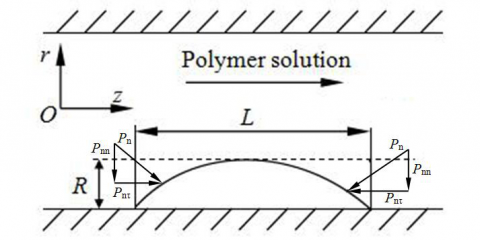Figure 1. Stress in normal and shear directions

For the polymer solution in Figure1, its constitutive equation under low Deborah number is as follows:

$T=2\left[\mu A+\lambda \frac{D A}{D t}\right]$     (3)

where T is the partial stress tensor; μ and λ are viscosity and relaxation time, respectively; A is the first-order Rivlin-Ericksen tensor; D/Dt is the simultaneous derivative. For convenience, expand the dimensionless form of equation (3). Hence, dimensionless constitutive equation in cylindrical coordinates is:

$T_{i j}=\vec{u}_{i, j}+\vec{u}_{j, i}+D e\left[\begin{array}{c}{u^{s} \frac{\partial}{\partial x^{s}}\left(\vec{u}_{i, j}+\vec{u}_{j, i}\right)+} \\ {\frac{1}{2} g^{s t}\left(\vec{u}_{t, j}-\vec{u}_{j, t}\right)\left(\vec{u}_{i, s}+\vec{u}_{s, i}\right)+} \\ {\frac{1}{2} g^{s t}\left(\vec{u}_{t, i}-\vec{u}_{i, t}\right)\left(\vec{u}_{j, s}+\vec{u}_{s, j}\right)}\end{array}\right]$        (4)

where $D e=u_{0} \lambda / R$;$u_{i, j}=\partial u_{i} / \partial x_{j}$; i, j, s and t= 1, 2, 3; x1= z; x2= r; x3= θ; gst is the metric tensor.

The dimensionless motion equations of the polymer solutions [23-25] are:

$-\frac{\partial p}{\partial r}+\frac{1}{r} \frac{\partial}{\partial r}\left(r T_{r r}\right)+\frac{\partial T_{z r}}{\partial z}-\frac{T_{\theta \theta}}{r}=\operatorname{Re}\left(\vec{u}_{r} \frac{\partial u_{r}}{\partial r}+\vec{u}_{z} \frac{\partial u_{r}}{\partial z}\right)$     (5)

$-\frac{\partial p}{\partial z}+\frac{1}{r} \frac{\partial}{\partial r}\left(r T_{r z}\right)+\frac{\partial T_{z r}}{\partial z}=\operatorname{Re}\left(\vec{u}_{r} \frac{\partial u_{z}}{\partial r}+\vec{u}_{z} \frac{\partial u_{z}}{\partial z}\right)$      (6)

where $R e=u_{0} \mathrm{d} R / \mu$;

The continuity equation is:

$\operatorname{div} \vec{u}=0$     (7)

Introduce the flow function Ψ. Then, the velocity component expressed by Ψ is:

$\vec{u}_{r}=-\frac{1}{r} \frac{\partial \Psi}{\partial z}, \vec{u}_{z}=\frac{1}{r} \frac{\partial \Psi}{\partial r}$     (8)

Assuming that the corrugated oil-water interface between along the z axis satisfies the following equation:

$r_{w}=\left[1-T \cos \left(2 \pi \frac{z}{L}\right)\right]$      (9)

and ur = uθ= 0 at the internal and external interfaces; Ψ=14π at the internal interface; Ψ=1π at the external interface.

Substitute boundary conditions into equations (3)~(9) to obtain the stress distribution of the polymer solution at different positions.

When the interface is curved, the control equations should be solved through coordinate conversion. Thus, introduce $y=r / r_{w}$and $\hat{z}=L / R$, focus on a cosine period where $10 \geq y \geq 1, L / R \geq \hat{z} \geq 0$, and solve it with the finite difference method.

3. Stress Analysis of Residual Oil on Lipophilic Rocks

3.1 Simplified model of 3D channels

The 3D channels were simplified into a cylinder with residual oil at the bottom. Figure 2(a) presents the simplified models of lipophilic, neutral wetting and hydrophilic flow channels, where BS1 is the inlet of the channels; BS2 is the outlet of the channels; BS3 is the interface between the polymer solution and rock; BS4 is the surface of residual oil; BS5 is the interface between rock and residual oil. The diameter is d=20μm for both the inlet and the outlet; the channel is 100μm in length; the residual oil lies in the bottom centre of the channel at the height of h=10μm; the length of the residual oil is 40μm in lipophilic channel, 20μm in neutral wetting channels and 10μm in hydrophilic channels. If the residual oil remains still, the flow of the polymer solution can be treated as a stationary problem. 3D calculations were performed on ANSYS Polyflow. Since the residual oil is located at the bottom of capillary micro-channels, the origin of coordinates was set at the centre of the residual oil to facilitate calculation. Therefore, a Cartesian coordinate system is adopted for this research. The contour of the residual oil was plotted separately to observe its shape (Figure 2(b)).

## 18_2.png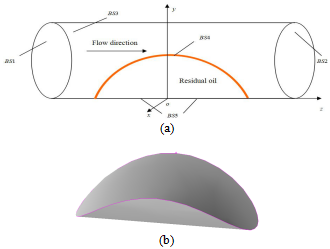Figure 2. 3D illustration of residual oil

3.2 Calculation of normal partial stress

Only the normal partial stress and horizontal stress difference were calculated, for the former reflects the distribution of residual oil, and the latter reflects the magnitude of the displacement force. In the 3D calculation model, the author figured out the variation patterns of the displacement force on the residual oil horizontal and vertical to the flow direction. Three planes were selected horizontal to the flow direction (x=0, x=3μm, x=6μm), and another three were selected vertical to the flow direction (and z=0, z=5μm, z=-5μm). The 6 planes result in the 6 intersected lines in Figure 3. The 3 horizontal lines are formed by planes x=0, x=3μm, x=6μm and the surface of the residual oil, and are denoted as 1#, 2#, 3# from the bottom. The 3 vertical lines are formed by planes z=-5μm, z=0, z=5μm and the surface of residual oil, and are denoted as 4#, 5#, 6# from the left.

## 3.png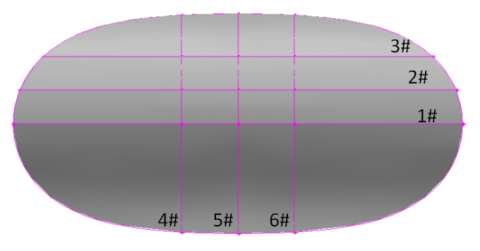Figure 3. Surface of residual oil

Figure 4 depicts the normal partial stress from the polymer solution on the residual oil along the 6 lines. In this chapter, the compressive stress is set as positive, and the tensile stress is set as negative.

## 18_1.png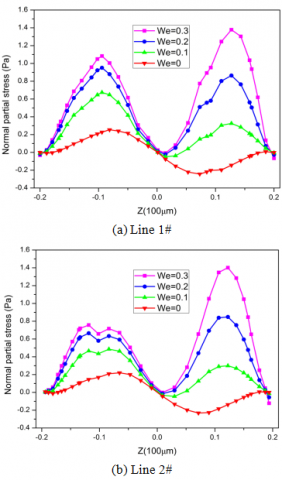## 18_cd.png## 18ef.pngFigure 4. Curves of normal partial stress

Note: The normal partial stress increases with Weissenberg number, leading to changes in distribution patterns. When We=0, the normal partial stress obeys asymmetric distribution; the residual oil is compressed in the upstream and tensioned in the downstream, creating a favourable condition for displacement; however, the force is too small to displace all the residual oil from the rock surface. With further increase in Weissenberg number, the asymmetric distribution gradually weakens. In this case, most of the residual oil is in compression, with only a minor portion tensioned near the centre. Such a stress distribution facilitates the local bulging or deformation of residual oil. The normal partial stress is negatively proportional to the distance from the centre, and is almost symmetrically distributed vertical to the flow direction.

The 3D normal partial stress was plotted in MATLAB to observe its distribution across the surface of residual oil (Figure 5).

## 5.pngFigure 5. 3D illustration of normal partial stress

3.3 Calculation of horizontal stress difference

As mentioned above, the three planes horizontal to the flow direction (x=0, x=3μm, x=6μm) form 3 lines (1#, 2#, 3#) on the surface of residual oil. The horizontal stress distribution along the 3 lines are shown in Figure 6.

It can be seen from Figure 6 that: when We=0, the horizontal stress difference decreases along the flow direction; with the increase in We, the horizontal stress difference first climbs up slowly to the peak before taking a deep plunge. The distribution indicates that the elastoviscous fluid has induced a shift in the extreme point of the residual oil. Moreover, the positive correlation between We, an indicator of elasticity, and the extreme point of the horizontal stress is attributable to the “memory” of the viscoelastic fluid. The extreme point is located at about 3/4 of the height of residual oil, where the residual oil is prone to bulging or deformation. If the deformation worsens and reaches the critical capillary number, the residual oil will burst into smaller scatted oil droplets. It is also inferred that the horizontal stress gets smaller the farther away from the centre of the residual oil.

4. Oil Displacement Visualization Experiment

4.1 Experiment apparatus and procedure

The existing experiment apparatuses cannot measure the exact stress state on the surface of the residual oil, wherefore the microscopic oil displacement visualization experiment was conducted to qualitatively analyse the deformation of the residual oil, which existed in different forms in micro pores. The oil displacement visualization apparatus can clearly reflect the residual oil distribution after polymer waterflooding, and accurately represent the micro displacement process, making it possible to compare polymer waterflooding with water flooding. Moreover, the apparatus also facilitates the theoretical analysis of the stress distribution and deformation of residual oil, and sheds new light on reliable stress and deformation analysis.

According to residual oil distribution after water flooding, the author observed the displacing efficiency of polymer waterflooding, and further analysed the distribution pattern and deformation of residual oil. As shown in Figure 7, the oil displacement visualization apparatus consists of a micro model, a displacement system, a lighting system, an image collecting system and image processing system. Specifically, the micro model is placed horizontally; the light box provides uniform lighting to ensure image quality; the image collecting system, involving a camera, a video recorder and a storage module, collects real-time images; the image processing system illustrates the static distribution of pores and oil, and calculates oil saturation and fractal dimensions of oil and pores. Furthermore, the pores for oil displacement were simulated by etched glass. The flow mode was set as constant flow rate in the displacing system. The images taken in oil displacement were collected and processed into numerical signals, and then analysed by the image processing system to reveal the micro features of the displacement of the surfactant system.

## 6.png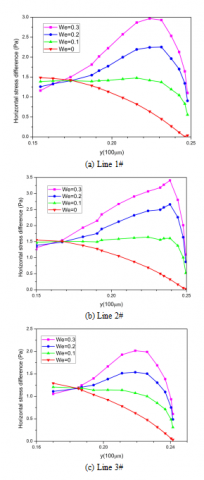Figure 6. Curves of horizontal stress distribution

## 7.png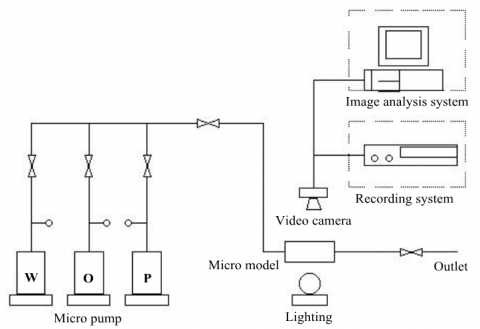Figure 7. Oil displacement visualization apparatus

Transparent glass-etched core samples (40mm×40mm) were made according to the structure of a flake from a real core sample. The experiment temperature was set to 45°C; the simulated oil is a mixture of crude oil and kerosene with a viscosity of 20mPa·s at 45°C; the HPAM was adopted as the polymer with a molecular weight of 19 million amu; the salinity of the test water was 508mg/L. The displacement process was simulated by the micro model in a thermostatic chamber. The deformation of the residual oil membrane was recorded to explore the effect on displacement efficiency. The experiment was conducted in the following steps: (1) vacuum the micro model and saturate it with oil; (2) displace the oil for 40min in the model with water at the flow rate of the water flooding process until no water is produced; (3) inject polymer solution at a flow rate of 1m/d, record the displacing process and observe the distribution of residual oil afterwards; (4) analyse the images and calculate the displacement efficiency under this polymer concentration; (5) clean the core.

4.2 Experiment apparatus and procedure

The displacement of the residual oil was observed clearly on the apparatus. The displacement effect improves with the increase in oil saturation. The mechanism of the displacement is similar to that of traditional waterflooding. It was also observed that the residual oil membrane deformed and broke in the experiment (Figure 8).

Under the micro driving force, the bulge of the oil mass moved forward, forming a new independent oil membrane. Then, the remaining part of the oil mass supplemented the original position of the bulge. The movement of the entire oil mass was not rear-driven. Instead, it was propelled by the fact that the oil behind the bulge must supplement the oil loss in the front, and form a new oil mass. The capillary force produced by the bulge of the new oil mass cancelled out the pressure gradient effect of the driving force. The oil behind the bulge may seem to be being dragged forward. Actually, its movement came from the readjustment of the capillary force distributed around the oil mass. The readjusted capillary force always offset the driving force, which also moved the residual oil mass forward.

## 8.png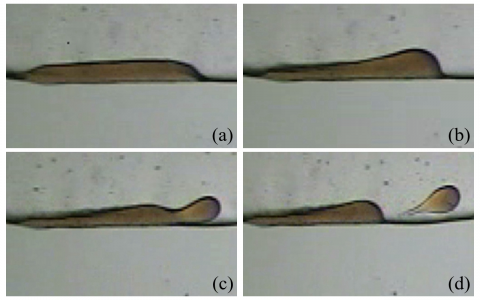Figure 8. Displacement of residual oil

As shown in Figure 8(b), the micro driving force from the polymer fluid first worked on the residual oil at the slope and bulge. As some of the residual oil moved from the slope to the bulge, the flow direction and velocity of the displacing fluid underwent greater changes, and the normal partial stress shown in Figure 4 was pushed up. As shown in Figure 6, the bulge tended to deform under the horizontal stress difference, and grew larger until it was separated from the oil mass. Then, it became an independent oil membrane that kept moving forward. The bulge always moved along the direction of fluid flow in the channel, because its movement was driven by the velocity variation of the displacing fluid. Most of the oil concentrated in the rear of the oil mass, and moved in random directions under the action of the capillary force. As shown in Figure 8, the main body of the oil mass moved sometimes, if not always, in the same direction with the bulge.

The inverse capillary force from the bulge cancelled out the driving force on the entire oil mass. The micro force only impacted the side of the oil mass near the centre of the channel, allowing the fluid to revolve under the oil mass surface. If the revolving rate was not constant, the oil mass would deform. The flow rate at a given point of the fluid was correlated to flow friction and the corresponding micro force. Since the viscosity is constant at any point of the fluid and the local capillary force is vertical to the flow direction in the oil mass, the flow friction relied on the thickness of the residual oil: the thicker the oil mass, the less the friction. When the other parameters were kept unchanged, it was discovered that the micro driving force increased with the angle between the fluid surface and the flow direction of the displacing fluid (the angle). Due to the initial thinness of the oil mass, there was a great friction force at the beginning. Thus, the micro force or the angle should be sufficiently large to ensure the flow rate within the oil mass. At the back, the oil mass grew thicker, producing less friction. Hence, the micro force and the angle gradually declined.

The displacing fluid was injected repeatedly to simulate the multiple displacement processes until the entire oil mass scattered into many tiny moving oil membranes (Figure8(d)), or the oil mass was too small for the micro driving force to accumulate enough oil and form an independent oil membrane with a decent diameter. At this point, the micro driving force achieved a phase balance with the capillary force on the oil mass, leaving a new, smaller and more stable oil mass behind. In the end, the oil structuration was increased, and the displacing efficiency was elevated.

5. Conclusions

The upper-convected Maxwell model was adopted to simulate the viscoelastic polymer solution. After establishing the flow equation of viscoelastic fluid in porous media and a 3D model of the displacement process, the author displaced the residual oil by the viscoelastic polymer solution in 3D micro-channels, recorded the normal partial stress and horizontal stress difference horizontal and vertical to the flow direction, and observed the deformation of the residual oil in the experiment. The conclusions are as follows:

(1) The increase in Weissenberg number leads to growth of the normal partial stress and variation in the distribution pattern. When Weissenberg number is high, the residual oil is mainly compressed with a small fraction tensioned near the centre, creating a favourable condition for deformation or local bulging. When We=0, the horizontal stress difference decreases along the flow direction; with the increase in We, the horizontal stress difference first climbs up slowly to the peak before taking a deep plunge. The Weissenberg number is positively correlated with horizontal stress difference, which is responsible for local bulging or deformation. If the deformation worsens and reaches the critical capillary number, the residual oil will burst into smaller scatted oil droplets.

(2) The deformation and breakage of the residual oil in the polymer waterflooding were observed through the microscopic displacement visualization experiment, and the stress state of the residual oil in the displacement process was qualitatively analysed. With numerical simulation, this research provides fresh insights into the stress state of the residual oil in displacement.

Acknowledgment

This work was supported by the National Science Foundation in China through grant No. 51374076 and University Nursing Program for Young Scholars with Creative Talents in Heilongjiang Province through grant No. UNPYSCT2016121.

Nomenclature
 A first order Rivlin-Ericksen tensor L length of residual oil, m N1 primary normal stress difference R height of residual oil, m T partial stress tensor We Weissenberg number Greek symbols λ relaxation time, s µ dynamic viscosity, kg. m-1.s-1 τ shear viscosity, Pa φ1 primary normal stress difference coefficient Ψ flow function
References

 Zhang L.J., Yue X.A. (2008). Displacement of polymer solution on residual oil trapped in dead ends, Journal of Central South University, Vol. 15, No. s1, pp. 84-87. DOI: 10.1007/S11771-008-320-4

 Hou J. (2007). Network modeling of residual oil displacement after polymer flooding, Journal of Petroleum Science & Engineering, Vol. 59, No. 3, pp. 321-332.

 Jamaloei B.Y., Kharrat R. (2010). Analysis of microscopic displacement mechanisms of dilute surfactant flooding in oil-wet and water-wet porous media, Transport in Porous Media, Vol. 81, No. 1, pp. 1-19.

 Shi S.M., Wei H.B., Li B., Huo D.K. (2013). Research on residual oil distribution regular of steam flooding after polymer flooding, Advanced Materials Research, Vol. 737, pp. 1440-1444.

 Chou T.H., Hong S.J., Sheng Y.J., Tsao H.K. (2012). Drops sitting on a tilted plate: receding and advancing pinning, Langmuir the Acs Journal of Surfaces & Colloids, Vol. 28, No. 11, pp. 5158-5166.

 Cristini V., Guido S., Alfani A. (2003). Drop breakup and fragment size distribution in shear flow, Journal of Rheology, Vol. 47, No. 5, pp. 1283-1298. DOI: 10.1122/1.1603240

 Davidson M.R., Cooper-White J.J. (2003). Numerical prediction of shear-thinning drop formation, Third International Conference on CFD in the Minerals and Process Industries CSIRO, Melbourne.

 Li X., Sarkar K. (2005). Effects of inertia on the rheology of a dilute emulsion of drops in shear, Journal of Rheology,Vol. 49, No. 6, pp. 1377-1394.

 Li X., Sarkar K. (2005). Drop dynamics in an oscillating extensional flow at finite Reynolds numbers, Physics of  fluids, Vol. 17, No. 2, pp. 177.

 Li X., Sarkar K. (2005). Negative normal stress elasticity of emulsions of viscous drops at finite inertia, Physical Review Letters, Vol. 95, No. 25, pp. 256001-1-4.

 Grand N.L., Daerr A., Limat L. (2005). Shape and motion of drops sliding down an inclined plane, Journal of Fluid Mechanics, Vol. 541, pp. 293-315.

 Megias-Alguacil D., Windhab E.J. (2006). Experimental study of drop deformation and breakup in a model multitoothed rotor-stator, Journal of Fluids Engineering, Vol. 128, No. 6, pp. 1289-1294. DOI: 10.1115/1.2354528

 Garstecki P., Fuerstman M.J., Stone H.A., Whitesides G.M. (2006). Formation of droplets and bubbles in a microfluidic T-junction-scaling and mechanism of break-up, Lab on A Chip, Vol. 6, No. 3, pp. 437-446. DOI: 10.1039/b510841a

 Van DG.S., Nisisako T., Schroën C.G., Van R.G., Boom R.M. (2006). Lattice Boltzmann simulation of droplet formation in a T-shaped microchannel, Journal of Colloid & Interface Science, Vol. 22, No. 9, pp. 4144-4152.

 Seevaratnam G., Michel O., Heng J., et.al. (2010). Laminar ﬂow deformation of a droplet adhering to a wall in a channel, Chemical Engineering Science, Vol. 65, No. 16, pp. 4523-4534.

 Chung C., Lee M., Char K., Ahn K.H., Lee S.J. (2010). Droplet dynamics passing through obstructions in confined microchannel flow, Microfluidics and Nanofluidics, Vol. 9, No. 6, pp. 1151-1163. DOI: 10.1007/s10404-010-0636-x

 Mulligan M.K., Rothstein J.P. (2011). The effect of conﬁnement-induced shear on drop deformation and breakup in microﬂuidic extensional ﬂows, Phsics of Fluids, Vol. 23, No. 2, pp. 022004-1-11.

 Tomas C., Bibin M.J., Samira D. (2012). Droplet breakup and viscosity-stratiﬁed ﬂows in microchannels, International Journal of Multiphase Flow, Vol. 39, pp. 29-36.

 Jie M.A., Hong R., Ding J., Yang J. (2005). Drop dynamics of the elastic fluids using Oldroyd-B model, Computers & Applied Chemistry, Vol. 22, No. 12, pp. 1173-1178.

 Barnes H.A., Hutton J.F., Walters K. (1989). An Introduction to Rheology, Elsevier Science & Technology, pp. 92-98.

 Aidaoui L., Lasbet Y.H., Loubar K. (2016). Numerical analysis of the parameters governing 3D laminar mixed convection flow in a rectangular channel with imposed wall flux density, International Journal of Heat and Technology, Vol. 34, No. 4, pp. 581-589. DOI: 10.18280/ijht.340405

 Shaw M.T. (2012). Introduction to Polymer Rheology, John Wiley, USA, pp. 83-89.

 Cui W.Z., Zhang X.T., Li Z.X., Li H., Liu Y. (2017). Three-dimensional numerical simulation of flow around combined pier based on detached eddy simulation at high Reynolds numbers, International Journal of Heat and Technology, Vol. 35, No. 1, pp. 91-96. DOI: 10.18280/ijht.350112

 Bird R.B. (1987). Dynamics of Polymeric Liquids, Wiley-Interscience, USA, pp. 125-131.

 Stergios Pilitsis. (1989). Calcalation of steady-state viscoelastic flow in an undulating tube, Journal of Non-Newtonian Fluid Mechanics, Vol. 31, No. 3, pp. 231-287.